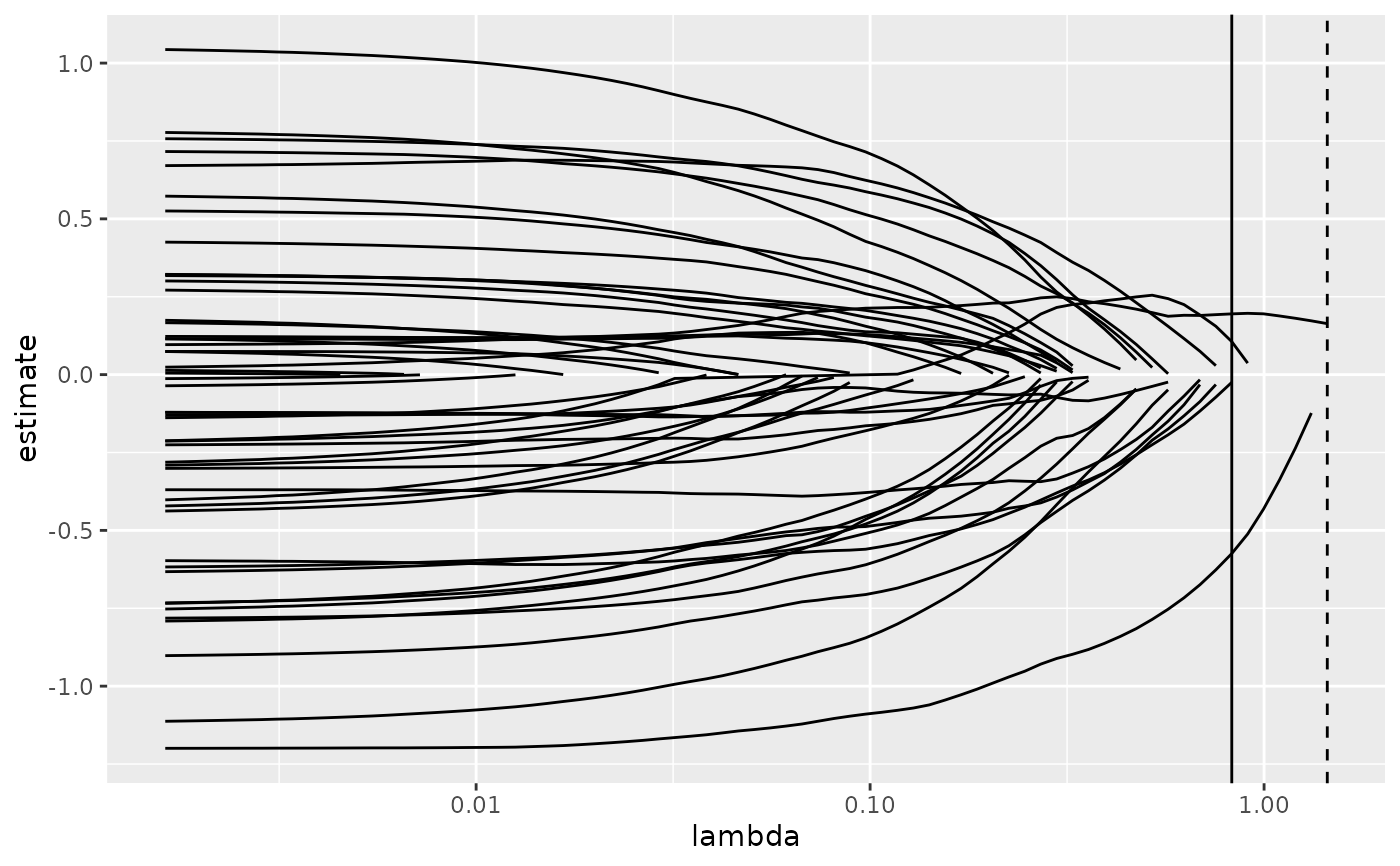Glance accepts a model object and returns a tibble::tibble() with exactly one row of model summaries. The summaries are typically goodness of fit measures, p-values for hypothesis tests on residuals, or model convergence information.

Glance never returns information from the original call to the modeling function. This includes the name of the modeling function or any arguments passed to the modeling function.

Glance does not calculate summary measures. Rather, it farms out these computations to appropriate methods and gathers the results together. Sometimes a goodness of fit measure will be undefined. In these cases the measure will be reported as NA.

Glance returns the same number of columns regardless of whether the model matrix is rank-deficient or not. If so, entries in columns that no longer have a well-defined value are filled in with an NA of the appropriate type.

## Usage

# S3 method for cv.glmnet
glance(x, ...)

## Arguments

x

A cv.glmnet object returned from glmnet::cv.glmnet().

...

Additional arguments. Not used. Needed to match generic signature only. Cautionary note: Misspelled arguments will be absorbed in ..., where they will be ignored. If the misspelled argument has a default value, the default value will be used. For example, if you pass conf.lvel = 0.9, all computation will proceed using conf.level = 0.95. Two exceptions here are:

• tidy() methods will warn when supplied an exponentiate argument if it will be ignored.

• augment() methods will warn when supplied a newdata argument if it will be ignored.

glance(), glmnet::cv.glmnet()

Other glmnet tidiers: glance.glmnet(), tidy.cv.glmnet(), tidy.glmnet()

## Value

A tibble::tibble() with exactly one row and columns:

lambda.1se

The value of the penalization parameter lambda that results in the sparsest model while remaining within one standard error of the minimum loss.

lambda.min

The value of the penalization parameter lambda that achieved minimum loss as estimated by cross validation.

nobs

Number of observations used.

## Examples


# load libraries for models and data
library(glmnet)

set.seed(27)

nobs <- 100
nvar <- 50
real <- 5

x <- matrix(rnorm(nobs * nvar), nobs, nvar)
beta <- c(rnorm(real, 0, 1), rep(0, nvar - real))
y <- c(t(beta) %*% t(x)) + rnorm(nvar, sd = 3)

cvfit1 <- cv.glmnet(x, y)

tidy(cvfit1)
#> # A tibble: 74 × 6
#>    lambda estimate std.error conf.low conf.high nzero
#>     <dbl>    <dbl>     <dbl>    <dbl>     <dbl> <int>
#>  1  1.45      17.4      2.28     15.1      19.7     0
#>  2  1.32      17.4      2.28     15.1      19.7     1
#>  3  1.20      17.2      2.22     15.0      19.5     1
#>  4  1.09      17.0      2.15     14.8      19.1     1
#>  5  0.997     16.8      2.09     14.7      18.9     1
#>  6  0.909     16.7      2.03     14.7      18.7     2
#>  7  0.828     16.7      1.99     14.7      18.6     3
#>  8  0.754     16.7      1.95     14.7      18.6     5
#>  9  0.687     16.8      1.93     14.8      18.7     7
#> 10  0.626     16.9      1.91     15.0      18.8     7
#> # ℹ 64 more rows
glance(cvfit1)
#> # A tibble: 1 × 3
#>   lambda.min lambda.1se  nobs
#>        <dbl>      <dbl> <int>
#> 1      0.828       1.45   100

library(ggplot2)

tidied_cv <- tidy(cvfit1)
glance_cv <- glance(cvfit1)

# plot of MSE as a function of lambda
g <- ggplot(tidied_cv, aes(lambda, estimate)) +
geom_line() +
scale_x_log10()
g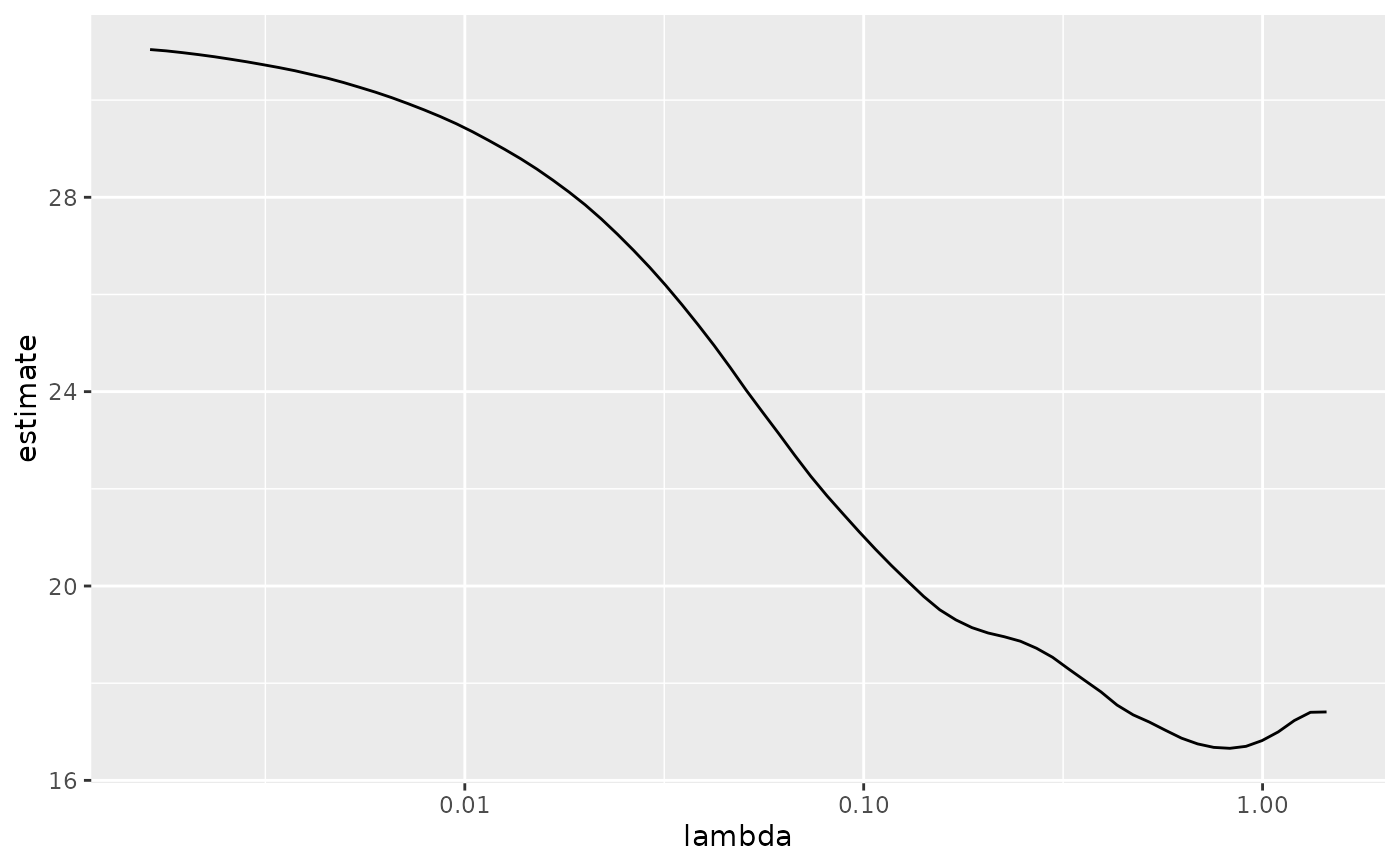# plot of MSE as a function of lambda with confidence ribbon
g <- g + geom_ribbon(aes(ymin = conf.low, ymax = conf.high), alpha = .25)
g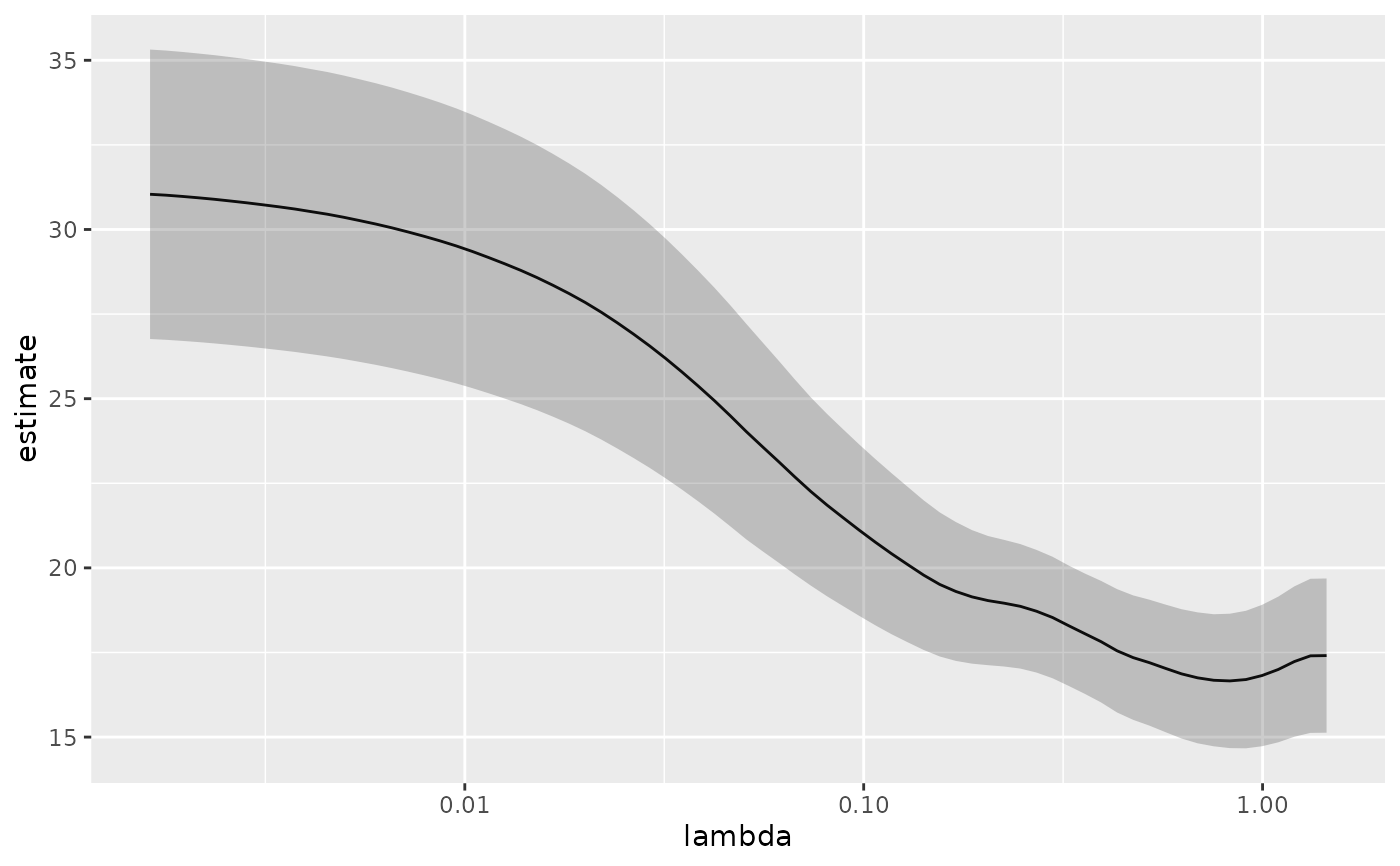# plot of MSE as a function of lambda with confidence ribbon and choices
# of minimum lambda marked
g <- g +
geom_vline(xintercept = glance_cv$lambda.min) + geom_vline(xintercept = glance_cv$lambda.1se, lty = 2)
g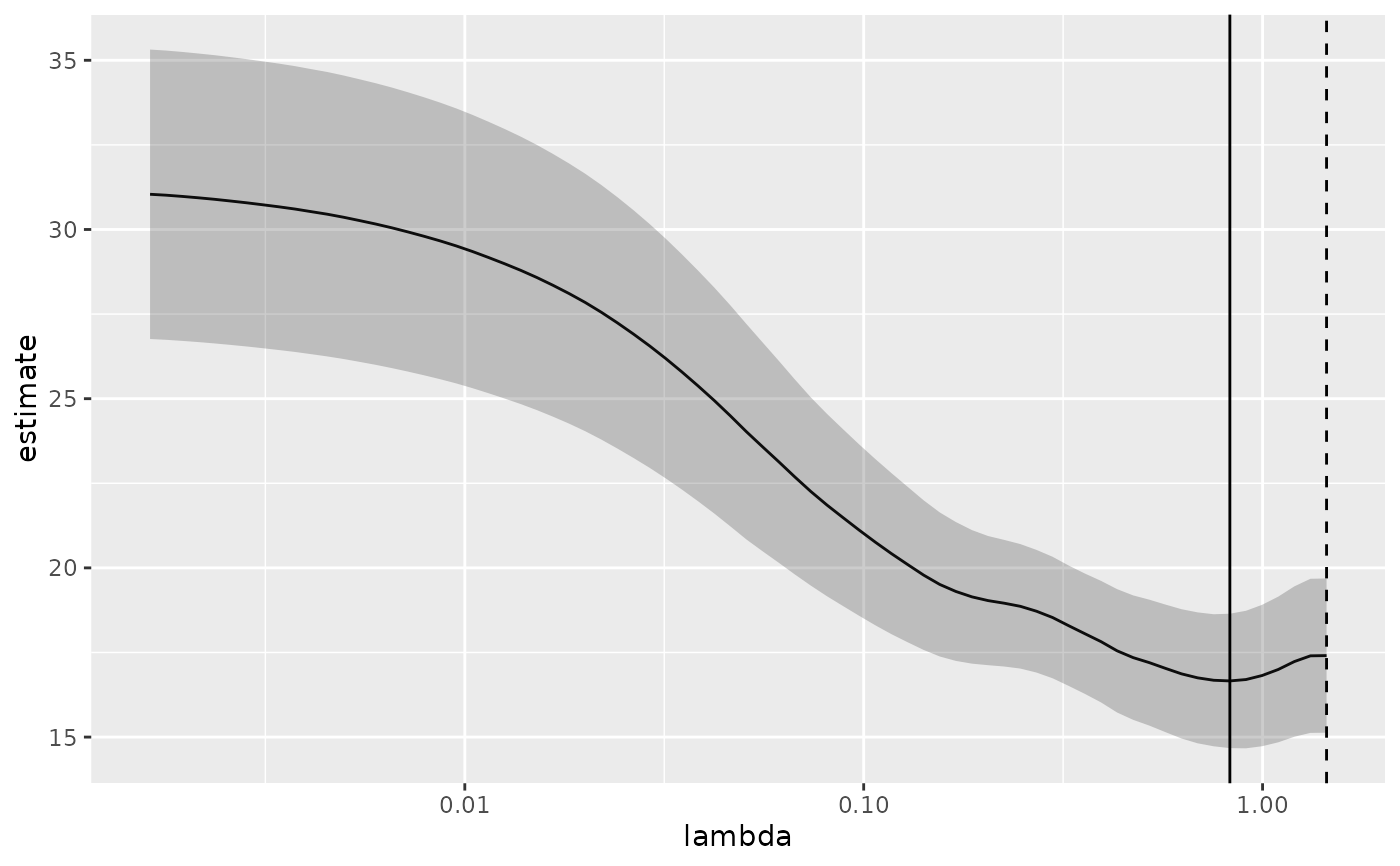# plot of number of zeros for each choice of lambda
ggplot(tidied_cv, aes(lambda, nzero)) +
geom_line() +
scale_x_log10()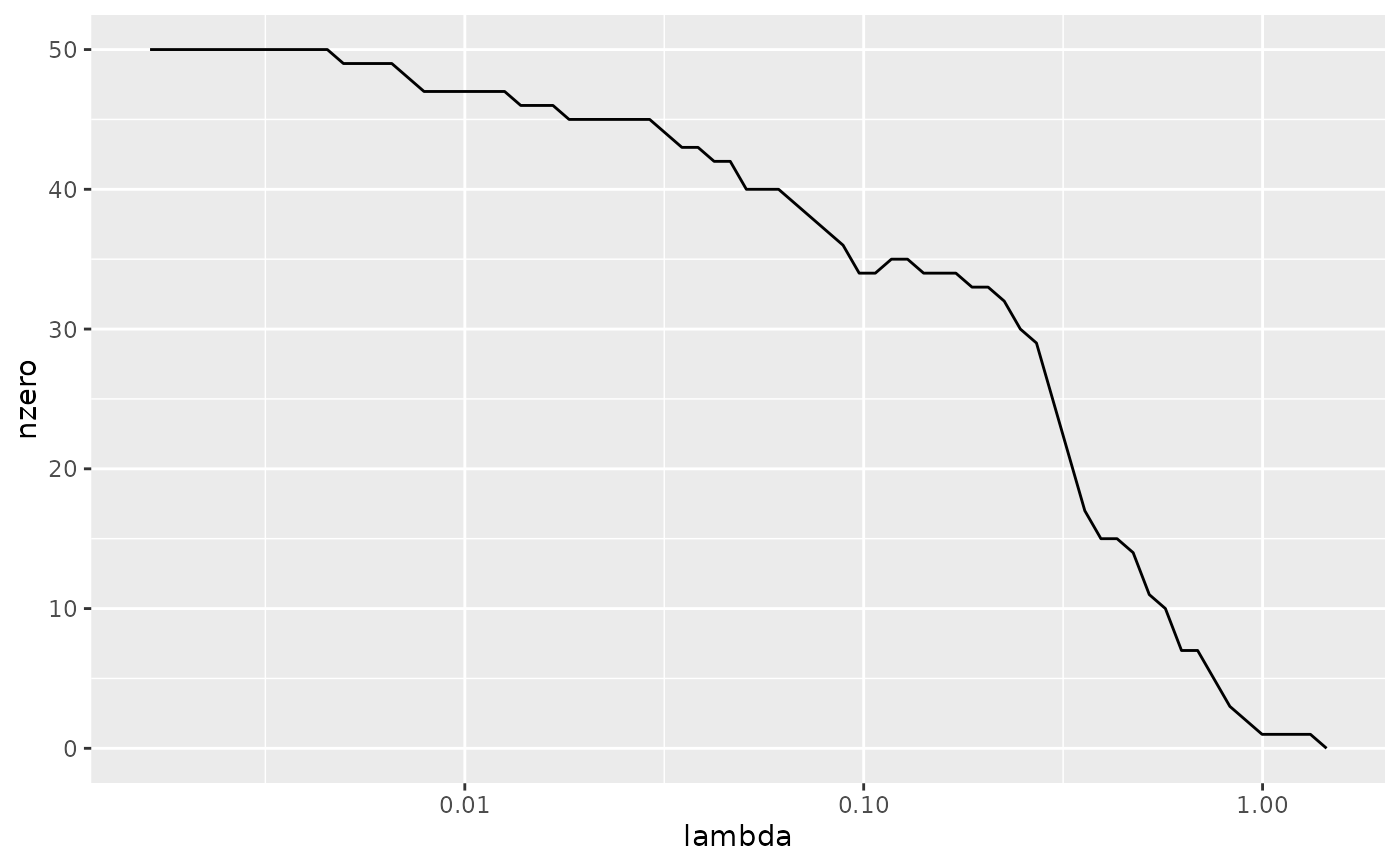# coefficient plot with min lambda shown
tidied <- tidy(cvfit1$glmnet.fit) ggplot(tidied, aes(lambda, estimate, group = term)) + scale_x_log10() + geom_line() + geom_vline(xintercept = glance_cv$lambda.min) +
geom_vline(xintercept = glance_cv\$lambda.1se, lty = 2)